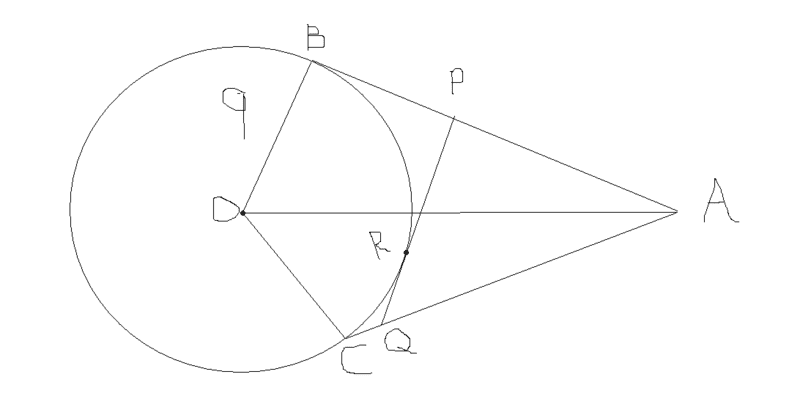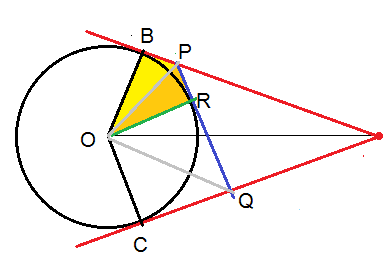# Goemetry perimeter problem

## Homework Statementthere is a circle with radius 9cm centred at o
segment oa is 15cm
ab, ca and pq are tangents to circle at points b,c and r respectively
find perimeter of triangle apq

## The Attempt at a Solution

i have solved the problem and got the right answer with two methods but i feel none of the methods is proper per say
method 1:
since the question is not imposing any restriction on the location of point r i assumed the perimeter is invariant with respect to r and hence let the segment pq fall on segment ca essentially the perimeter of two triangles is now just the two times the length of ac
ac is 12 cm by pythagoras hence the perimeter of triangle is 24 cm
a really crude method really dont think it is correct
method 2:
once with the assumption that the perimeter is invariant with respect to r
i shifted r right up to the point where da intersects the circle
then the triangle rpa is similar to triangle boa then rp is 9/2 and pa is 7.5 cm
then perimeter is once again 15 + 9 = 24 cm
however i feel my assumption is wrong and the question can be solved without the assumption so someone please help? thanks

ehild
Homework Helper

## Homework Statement

View attachment 205891
there is a circle with radius 9cm centred at o
segment oa is 15cm
ab, ca and pq are tangents to circle at points b,c and r respectively
find perimeter of triangle apq

## The Attempt at a Solution

i have solved the problem and got the right answer with two methods but i feel none of the methods is proper per say
method 1:
since the question is not imposing any restriction on the location of point r i assumed the perimeter is invariant with respect to r and hence let the segment pq fall on segment ca essentially the perimeter of two triangles is now just the two times the length of ac
ac is 12 cm by pythagoras hence the perimeter of triangle is 24 cm
a really crude method really dont think it is correct
method 2:
once with the assumption that the perimeter is invariant with respect to r
i shifted r right up to the point where da intersects the circle
then the triangle rpa is similar to triangle boa then rp is 9/2 and pa is 7.5 cm
then perimeter is once again 15 + 9 = 24 cm
however i feel my assumption is wrong and the question can be solved without the assumption so someone please help? thanksHow are the segments BP and PR related? The same with RQ and CQ?

•timetraveller123
BP = BR and RQ = CQ
and i also note that triangle PRO is similar to ORQ
ok that is smart thanks
perimeter = PA + PQ + AQ = BA - BP + CA - CQ + PQ = BA + CA- PQ + PQ = 24 thanks

ehild
Homework Helper
BP = BR and RQ = CQ
and i also note that triangle PRO is similar to ORQ
Are they??
ok that is smart thanks
perimeter = PA + PQ + AQ = BA - BP + CA - CQ + PQ = BA + CA- PQ + PQ = 24 thanks
It was simple, was not it?•timetraveller123
wait no they are not sorry my bad

yup it was simple